# Odd numbers

The sum of four consecutive odd numbers is 1048. Find those numbers ...

a =  259
b =  261
c =  263
d =  265

### Step-by-step explanation:

a+b+c+d=1048
b=a+2
c=b+2
d=c+2

a+b+c+d = 1048
a-b = -2
b-c = -2
c-d = -2

a = 259
b = 261
c = 263
d = 265

Our linear equations calculator calculates it.Did you find an error or inaccuracy? Feel free to write us. Thank you!

Showing 1 comment:Math student
Nobody taught me that in school so this is unclear to meTips to related online calculators
Do you have a linear equation or system of equations and looking for its solution? Or do you have a quadratic equation?

## Related math problems and questions:

• Unknown numbersThe sum of two consecutive natural numbers and their triple is 92. Find these numbers.
• Have solutionThe sum of four consecutive even numbers is 92. Determine these numbers.
• The sumThe sum of five consecutive odd numbers is 75. Find out the sum of the second and fourth of them.
• Real estate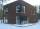The residential house has three entrances numbered by odd numbers arithmetic progression. The sum of the two numbers on the corner entrances is 50. Calculate the highest of these three numbers.
• Even / odd numbers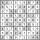a / Using variable n write two consecutive odd numbers. b / the sum of three consecutive odd numbers is 333. What are this numbers?
• Sum of odd numbersFind the sum of all odd integers from 13 to 781.
• Three ints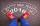The sum of three consecutive integers is 2016. What numbers are they?
• Sum of sevenThe sum of seven consecutive odd natural numbers is 119. Determine the smallest of them.
• Sum of four numbers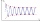The sum of four consecutive natural numbers is 114. Find them.
• AM of three numbersThe number 2010 can be written as the sum of 3 consecutive natural numbers. Determine the arithmetic mean of these numbers.
• Two numbers 7The sum of two consecutive even numbers is 30. Find the numbers.
• Find twoFind two consecutive natural numbers whose product is 1 larger than their sum. Searched numbers expressed by a fraction whose numerator is the difference between these numbers and the denominator is their sum.
• Find the sumFind the sum of all natural numbers from 1 and 100, which are divisible by 2 or 5
• Consecutive numbersSum of ten consecutive numbers is 105. Determine these numbers (write first and last).
• The sum 2The sum of five consecutive even integers is 150. Find the largest of the five integers. A.28 B.30 C.34 D.54 Show your solution and explain your answer.
• All pairs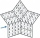Determine all pairs (m, n) of natural numbers for which is true: m s (n) = n s (m) = 70, where s (a) denotes the digit sum of the natural number a.
• Sum and ratioThe sum and ratio of two numbers is equal to 10. Which numbers are they?